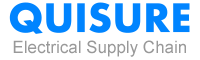My cart

Shopping cart is empty.

#HomePostsFAQCommon Question Answer of Current Transformer

## Common Question Answer of Current Transformer

From: Quisure2019-09-27

## 1. What are CT and PT in transformer?

CT means current transformers, which main are used in clamp meters to measure current. PT means potential transformer, which main are used in high voltage. Sometimes names transformer Voltage.

## 2. What is difference between CT and PT?

Both are instrumentation transformers. CT measures current PT measures voltage. CT is used in clamp meters. PTs measure high voltages. They both bring the measured values down to a standard of 5 ampere or 110 Voltage.

## 3. What is current transformer burden?

The secondary load of a current transformer is usually called the burden or Current Transformer Ratio, to distinguish it from the load of the circuit whose current is being measured. The burden, in a CT is the impedance presented to its secondary winding.

## 4. What is current transformer ratio?

Current transformers are transformers used to step down the current level of high-power transmission systems to measure or monitor the current levels. As a consequence, we use CTs to step down the current levels to measure it.

The CT ratio is the ratio of primary current input to secondary current output at full load. For examples, a CT with a ratio of 500:5 is rated for 500 primary amps at full load and will produce 5 amps of secondary current when 500 amps flow through the primary.

## 5. Why do we use current transformer?

A current transformer (CT) is a type of transformer that is used to reduce or multiply an alternating current (AC). It produces a current in its secondary which is proportional to the current in its primary. Engineers can calculate the primary current value by the secondary current and the current transformer ratio.